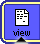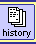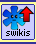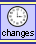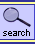## CLOS で bank-account

CLOS では、(DEFCLASS <class-to-def> (<superclass> ...) (slot-spec ...)) でクラスの定義ができるのですが、起動するメソッドの選択には総称関数という仕組みが用いられるため、ここで定義するのはスロット（インスタンス変数に相当）のみとなります。ただ、:accessor というオプションを付けることで、Ruby の attr のようなこと、つまり、ゲッター（や、値設定式中で使用できる関数）として使える総称関数を自動的に登録してくれるので、ごく基本的なオブジェクトの定義はこれだけ済ますことができます（そうでなくとも、スロットアクセスのための関数は別に用意されているので、それを使う手もあります）。

メソッドは別に関数として定義し（正確には、いったん関数を作り、それを総称関数として登録する２ステップ。DEFMETHOD は未登録の場合にこれを自動でやってくれるマクロ）、クラスに内包するようなことはしません（オブジェクト指向にも考え方やモデル、メタファがいろいろあります）。総称関数の起動は、引数の型の組み合わせで決定されます。

なぜか、引数の数の違う同名関数を登録することができないので、下の例ではセッターだけ名前を変えてあります。--sumim

```(defclass bank-account ()
((dollars :accessor dollars
:initform 0
:initarg :dollars)))

(defmethod set-dollars ((self bank-account) (x number))
(setf (dollars self) x))

(defmethod deposit ((self bank-account) (x number))
(set-dollars self (+ (dollars self) x)))

(defmethod withdraw ((self bank-account) (x number))
(set-dollars self (max 0 (- (dollars self) x))))

(setq my-account (make-instance 'bank-account :dollars 200))

(dollars my-account)
==> 200
(deposit my-account 50)
==> 250
(withdraw my-account 100)
==> 150
(withdraw my-account 200)
==> 0

(defclass stock-account (bank-account)
((num-shares :accessor num-shares
:initform 0
:initarg :num-shares)
(price-per-share  :accessor price-per-share
:initform 30
:initarg :price-per-share)))

(defmethod set-num-shares ((self stock-account) (x number))
(setf (num-shares self) x))

(defmethod set-price-per-share ((self stock-account) (x number))
(setf (price-per-share self) x))

(defmethod set-dollars ((self stock-account) (x number))
(set-num-shares self (/ (float x) (price-per-share self)))
(dollars self))

(defmethod dollars ((self stock-account))
(* (num-shares self) (price-per-share self)))

(setq my-stock (make-instance 'stock-account :num-shares 10))

(dollars my-stock)
==> 300
(set-dollars my-stock 600)
==> 600.0
(deposit my-stock 60)
==> 660.0
(num-shares my-stock)
==> 22.0
(withdraw my-stock 120)
==> 540.0
(num-shares my-stock)
==> 18.0```
--sumim

このページを編集 (2604 bytes)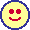以下の 3 ページから参照されています。